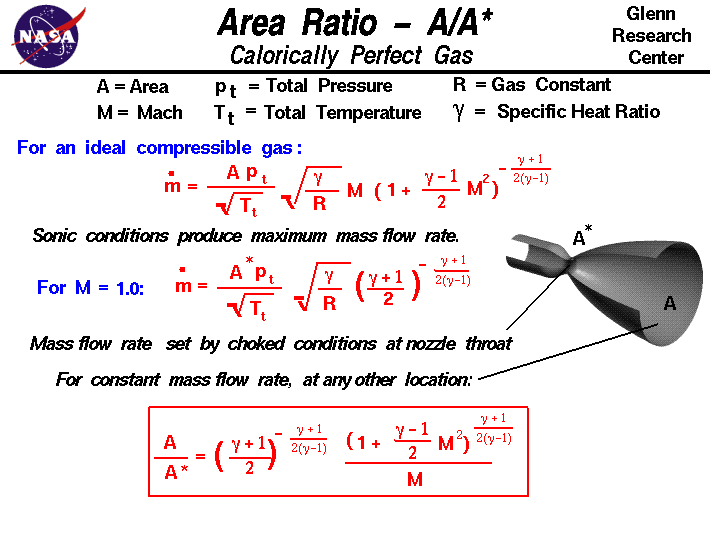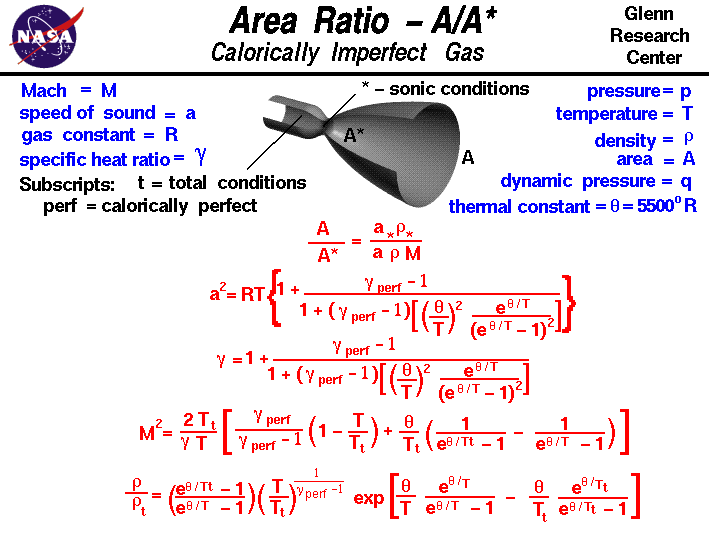+ Text Only Site
+ Non-Flash Version
+ Contact GlennThe conservation of mass is a fundamental concept of physics. Within some problem domain, the amount of mass remains constant; mass is neither created or destroyed. The mass of any object is simply the volume that the object occupies times the density of the object. For a fluid (a liquid or a gas) the density, volume, and shape of the object can all change within the domain with time and mass can move through the domain. The conservation of mass (continuity) tells us that the mass flow rate mdot through a tube is a constant. If we include compressibility effects for high speed flow through a duct, we find that the mass flow rate depends on the flow area A, total pressure pt and temperature Tt of the flow, the Mach number M, the ratio of specific heats of the gas gam, and the gas constant R: mdot = (A * pt/sqrt[Tt]) * sqrt(gam/R) * M * [1 + M^2 * (gam-1)/2]^-[(gam+1)/(gam-1)/2] During a flow process, like pushing a gas through a tube, the state of the thermodynamic variables of the gas can change. If the specific heat capacity is a constant value, the gas is said to be calorically perfect and if the specific heat capacity changes, the gas is said to be calorically imperfect. At subsonic and low supersonic Mach numbers, air is calorically perfect. But under low hypersonic flow conditions or high total temperature conditions, air is calorically imperfect. Derived flow variables, like the speed of sound and the isentropic flow relations are slightly different for a calorically imperfect gas than the conditions predicted for a calorically perfect gas because some of the energy of the flow excites the vibrational modes of the diatomic molecules of nitrogen and oxygen in the air. The continuity equation can be easily integrated to give the mass flow rate equation for a calorically perfect gas, as shown above. The procedure for a calorically imperfect gas is more difficult, as described below. The compressibility effects on mass flow rate have some unexpected results. We can increase the mass flow through a tube by increasing the area, increasing the total pressure, or decreasing the total temperature. But the effect of increasing velocity (Mach number) is a little harder to figure out. If we were to fix the area, total pressure and temperature, and graph the variation of mass flow rate with Mach number, we would find that a limiting maximum value occurs at Mach number equal to one. Using calculus, we can mathematically determine the same result: there is a maximum airflow limit that occurs when the Mach number is equal to one. The limiting of the mass flow rate is called choking of the flow. The value of the mass flow rate at choked conditions is given by: mdot = (A* * pt/sqrt[Tt]) * sqrt(gam/R) * [(gam+1)/2]^-[(gam+1)/(gam-1)/2] Mach number equal to one is called a sonic condition because the velocity is equal to the speed of sound and we denote the area for the sonic condition by "A*". If we have a tube with changing area, like the nozzle shown on the slide, the maximum mass flow rate through the system occurs when the flow is choked at the smallest area. This location is called the throat of the nozzle. The conservation of mass specifies that the mass flow rate through a nozzle is a constant. If no heat is added, and there are no pressure losses in the nozzle, the total pressure and temperature are also constant. By substituting the sonic conditions into the mass flow rate equation, and doing some algebra, we can relate the Mach number M at any location in the nozzle to the ratio between the area A at that location and the area of the throat A*. The resulting equation is shown in the box at the bottom of the slide. A / A* = {[(gam+1)/2]^-[(gam+1)/(gam-1)/2]} / M * [1 + M^2 * (gam-1)/2]^[(gam+1)/(gam-1)/2] For the calorically imperfect gas, we can not derive a simple equation for area ratio. The procedure to determine the area ratio is shown on this slide:From the continuity equation: A / A* = [ a* (rho*) ] / [ a * rho * M] where a* is the speed of sound at the sonic condition, rho* is the density at the sonic condition, a is the speed of sound at the exit, and rho is the density at the exit. Mathematical models based on a simple harmonic vibrator have been developed for the calorically imperfect gas. The details of the analysis were given by Eggars in NACA Report 959. A synopsis of the report is included in NACA Report 1135. To a first order approximation, the equation for the speed of sound for a calorically imperfect gas is given by: a^2 = R * T * {1 + (gamma - 1) / ( 1 + (gamma-1) * [(theta/T)^2 * e^(theta/T) /(e^(theta/T) -1)^2]) } where gamma is the ratio of specific heats for a perfect gas and theta is a thermal constant equal to 5500 degrees Rankine. The relation for the total temperature is given as: M^2 = (2 (Tt/T) / gam) * [(gamma/(gamma-1) * (1 - T/Tt) + (theta/Tt) * (1/(e^theta/Tt -1) - 1/(e^theta/T -1)] where gam is the ratio of specific heats including a correction for the vibrational modes: gam = 1 + (gamma - 1) / ( 1 + (gamma-1) * [(theta/T)^2 * e^(theta/T) /(e^(theta/T) -1)^2]) The equation relating Mach number and total temperature must be solved iteratively to obtain a value for the total temperature. Having the total temperature ratio,the relations between density and total temperature is: rho/rhot = [(e^(theta/Tt) - 1)/(e^(theta/T) - 1)] * [(T/Tt)^(1/(gamma-1))] * exp[(theta/T) * (e^(theta/T)/(e^(theta/T) -1)) - (theta/Tt) * (e^(theta/Tt)/(e^(theta/Tt) -1)) ] where rho/rhot is the ratio of the static density to the total density. This set of equations must be solved iteratively to determine the value for the area ratio. Here is a Java program that solves the area ratio equation. This page shows an interactive Java applet to learn how isentropic flows behave by varying the individual flow variables. By default, the program Input Variable is the Mach number of the flow. Since the area ratio depends only on the Mach number and ratio of specific heats, the program can calculate the value of the area ratio and display the results on the right side of the output variables. You can also have the program solve for the Mach number that produces a desired value of area ratio. Using the choice button labeled Input Variable, select "Area Ratio - A/A*". Next to the selection, you then type in a value for A/A*. When you hit the red COMPUTE button, the output values change. The area ratio is double valued; for the same area ratio, there is a subsonic and a supersonic solution. The choice button at the right top selects the solution that is presented. If you are an experienced user of this calculator, you can use a sleek version of the program which loads faster on your computer and does not include these instructions. You can also download your own copy of the program to run off-line by clicking on this button: Considering a rocket nozzle, we can set the mass flow rate by setting the area of the throat. And we can set the exit Mach number by setting the area ratio of the exit to the throat. Using the isentropic relations, we can determine the pressure and temperature at the exit of the nozzle. And from the Mach number and temperature we can determine the exit velocity. If we consider the rocket thrust equation, we have now determined all the values necessary to determine the thrust of the rocket. You can explore the operation of a nozzle with our interactive thrust simulator and design your own rockets! Activities: Guided Tours Navigation ..Beginner's Guide Home Page+ Inspector General Hotline + Equal Employment Opportunity Data Posted Pursuant to the No Fear Act + Budgets, Strategic Plans and Accountability Reports + Freedom of Information Act + The President's Management Agenda + NASA Privacy Statement, Disclaimer, and Accessibility CertificationEditor: Tom Benson NASA Official: Tom Benson Last Updated: May 07 2021 + Contact Glenn